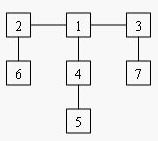﻿ 【POJ 1655 Balancing Act】 stdKonjac的小站

## 【POJ 1655 Balancing Act】

### Description

Consider a tree T with N (1 <= N <= 20,000) nodes numbered 1…N. Deleting any node from the tree yields a forest: a collection of one or more trees. Define the balance of a node to be the size of the largest tree in the forest T created by deleting that node from T.
For example, consider the tree:Deleting node 4 yields two trees whose member nodes are {5} and {1,2,3,6,7}. The larger of these two trees has five nodes, thus the balance of node 4 is five. Deleting node 1 yields a forest of three trees of equal size: {2,6}, {3,7}, and {4,5}. Each of these trees has two nodes, so the balance of node 1 is two.

For each input tree, calculate the node that has the minimum balance. If multiple nodes have equal balance, output the one with the lowest number.

### Input

The first line of input contains a single integer t (1 <= t <= 20), the number of test cases. The first line of each test case contains an integer N (1 <= N <= 20,000), the number of congruence. The next N-1 lines each contains two space-separated node numbers that are the endpoints of an edge in the tree. No edge will be listed twice, and all edges will be listed.

### Output

For each test case, print a line containing two integers, the number of the node with minimum balance and the balance of that node.

### Sample Output### 留下你的评论

*评论支持代码高亮<pre class="prettyprint linenums">代码</pre>